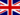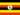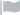Para uma experiência de aprendizado mais agradável, recomendamos que você estude a versão republicada amigável deste curso.

- or -

Continue studying this course

# Como calcular a ampliação

• Nota de Estudos
• Rever TópicosAngela A. 2 0 Each lens has a magnifcation factor. The Eye piece is x10 and the Objective lens is x40. To calculate an objects magnification is to multiply the Eye piece and the objective lens, this is equal to x400 magnification.Angela A. 0 0 why is this not in addition but in multiplication.Odongo M. 0 0 Biology -> How to calculate magnification How to calculate magnificationSusan G. 0 0 Each lens has a magnification factor. The object lens has x 40. The eyepiece on its own is x 10. This makes the magnification of thee microscope is 400.Hossam E. 0 0 niceDon M. 0 0 When calculating magnification is there a way to magnify without straining visual?### Você recebeu uma nova notificação

Clique aqui para visualizar todos eles